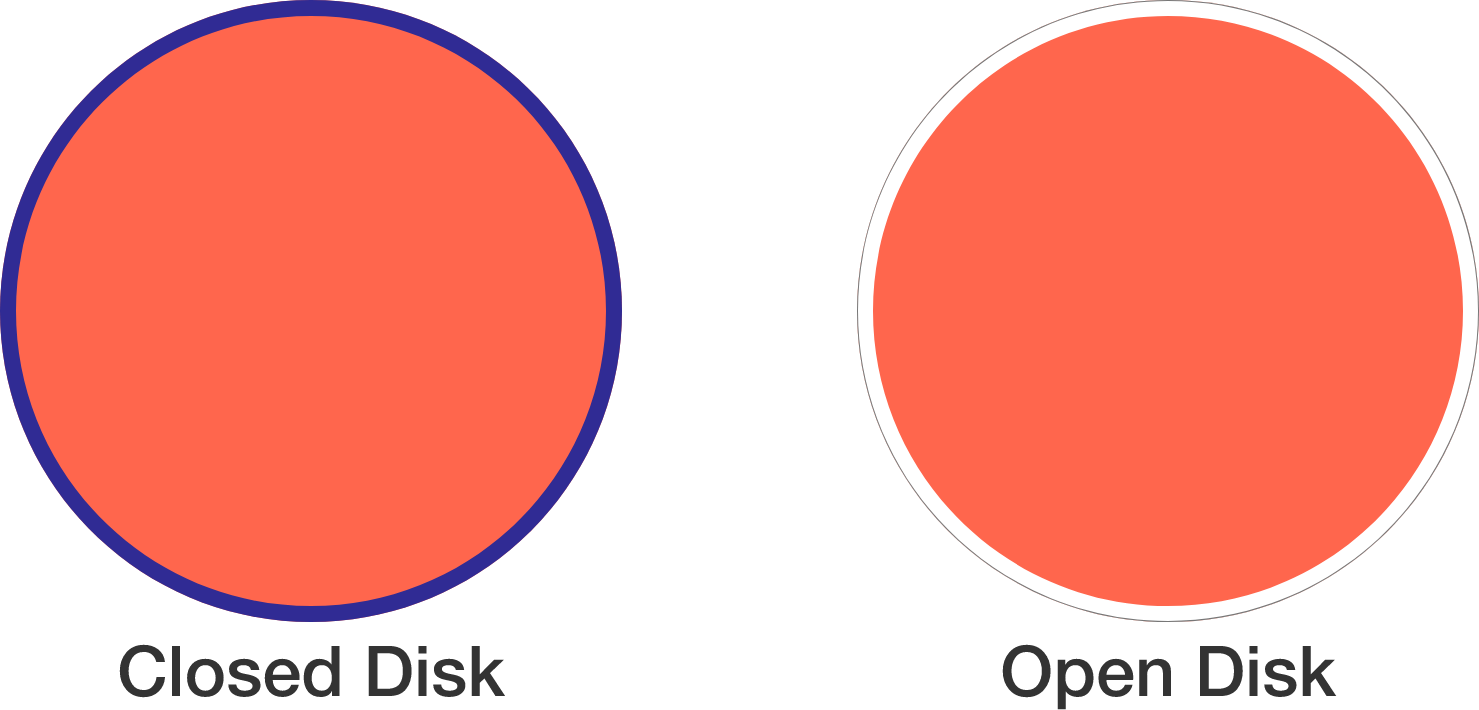# Coffee without the Cup

Calculus Level 3

Suppose $f$ is a continuous function that maps the closed unit disk on $\mathbb{R}^2$ to itself. Then, Brouwer's fixed-point theorem tells us that there is a fixed point $x_0$ in the closed disk which is mapped to itself, i.e. $f(x_0) = x_0$.In a similar spirit, let $g$ be a continuous function that maps the open unit disk to itself. Must $g$ have a fixed point?

×